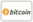Bookcover of Nonlinear Euler-Poisson-Darboux Equations
Booktitle:

# Nonlinear Euler-Poisson-Darboux Equations

## Initial Value Problems and Exact Solutions

LAP LAMBERT Academic Publishing (2011-03-30 )eligible for voucher
ISBN-13:

### 978-3-8443-2036-7

ISBN-10:
3844320369
EAN:
9783844320367
Book language:
English
Blurb/Shorttext:
This book is devoted to study multidimensional linear and nonlinear partial differential equations. Among several methods to deal with higher dimensional linear partial differential equations, the elegant method of Spherical Means has spacial importance since this method reduces the higher dimensional equations to the one dimensional radial equations of Euler-Poisson-Darboux type which are well studied. Although this method is applicable only to the linear differential equations, by some special transformations, like the Cole-Hopf transformation and the Backlaund transformation, exact solutions of multidimensional nonlinear partial differential equations of the Spherical Liouville, Sine- Gordon and Burgers type are constructed.
Publishing house:
Website:
https://www.lap-publishing.com/
By (author) :
Barış Ateş, Oktay K. Pashaev
Number of pages:
84
Published at:
2011-03-30
Stock:
Available
Category:
Mathematics
Price:
49.00 €
Keywords:
Euler-Poisson-Darboux Equation, Spherical Means, Nonlinear Partial Differential Equations, Multidimensional Wave Equation, Liouville Equation, Spherical Liouville Equation, Sine Gordon Equation, Spherical Sine Gordon Equation, Multidimensional Heat equation, Burgers Equation, Spherical Burgers equation., Nonlinear Partial Differential Equations### Categories